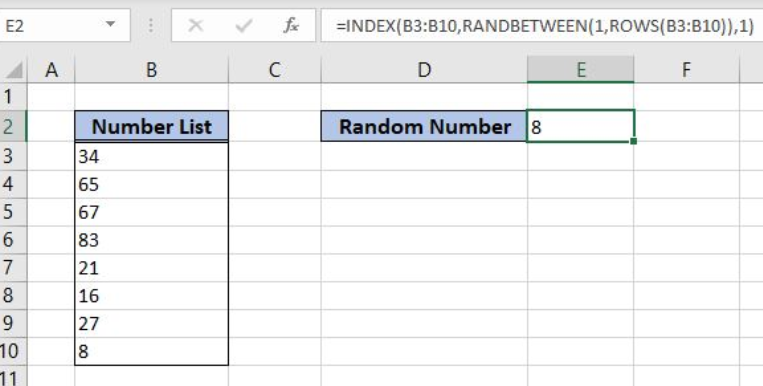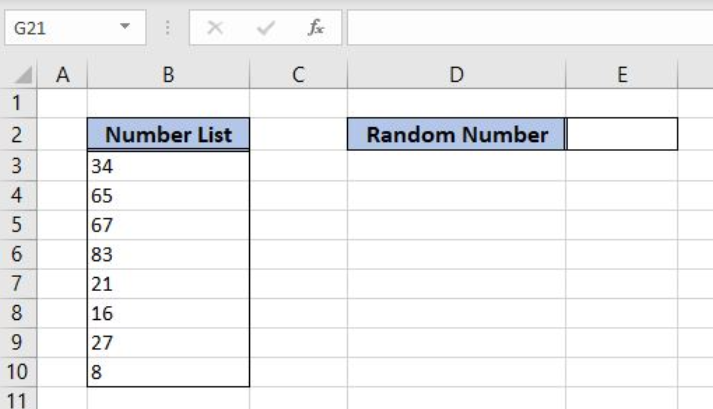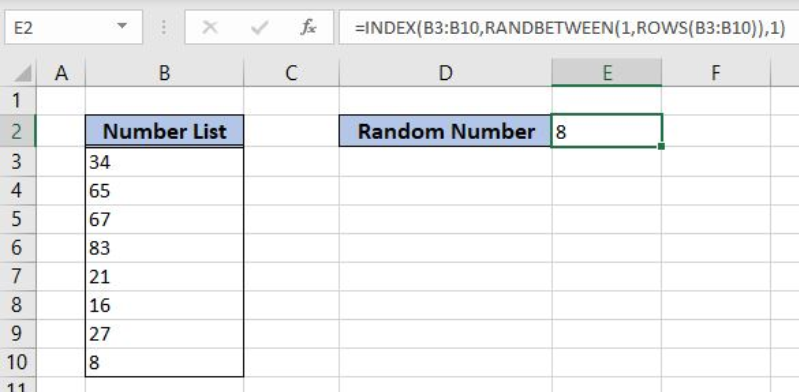Get instant live expert help with Excel or Google Sheets“My Excelchat expert helped me in less than 20 minutes, saving me what would have been 5 hours of work!”

#### Post your problem and you’ll get expert help in seconds.

Your message must be at least 40 characters
Our professional experts are available now. Your privacy is guaranteed.

# Learn How to Find a Random Number from a List in Excel

Excel allows us to get a random number from a list using the INDEX, RANDBETWEEN and ROWS functions. This step by step tutorial will assist all levels of Excel users to learn how to find a random number from a list in Excel.Figure 1. The final result of the formula

## Syntax of the INDEX formula

`=INDEX(array, row_num, [column_num])`

The parameters of the INDEX function are:

• array – a list of values where we want to find a value from a certain position
• row_num – a row from which we want to get a value
• [column_num] – a column from which we want to get a value. It’s a non-mandatory parameter and if it’s omitted it’s by default 1.

## Syntax of the RANDBETWEEN formula

`=RANDBETWEEN(bottom, top)`

The parameters of the RANDBETWEEN function are:

• bottom – a value from which we want to get a random value
• top – a value to which we want to get a random value

## Syntax of the ROWS formula

`=ROWS(array)`

The parameter of the ROWS function is:

• array – an array for which we want to get the total number of rows.

## Setting up Our Data for the Functions

In column B (“Number List”), we have the list of numbers where we want to find the random number. The result will be in the cell E2.Figure 2. Data that we will use in the example

## Get a Random Number from the List

In the cell E2, we want to get the random number from the list of names in the range B3:B10.

The formula looks like:

`=INDEX(B3:B10, RANDBETWEEN(1, ROWS(B3:B10)), 1)`

The array is B3:B10. The row_num is `RANDBETWEEN(1, ROWS(B3:B10))`, while the column_num is 1.

To apply the formula, we need to follow these steps:

• Select cell E2 and click on it
• Insert the formula: `=INDEX(B3:B10, RANDBETWEEN(1, ROWS(B3:B10)), 1)`
• Press enter.Figure 3. Using the formula to get a random name from the list

The INDEX function searches the B3:B10 range. The row_num is the result of the RANDBETWEEN function, which returns a random number between 1 and 8. In this example, this function returned 8, so the INDEX returns the value from the third row. Therefore, the result of the formula in the cell E2 is 8.

Most of the time, the problem you will need to solve will be more complex than a simple application of a formula or function. If you want to save hours of research and frustration, try our live Excelchat service! Our Excel Experts are available 24/7 to answer any Excel question you may have. We guarantee a connection within 30 seconds and a customized solution within 20 minutes.

Solution examplesI need help on index and match where the return is not correct
Solved by B. B. in 20 minsI have a database extract that is showing the same clients multiple times in the list. How can I write a formula in excel to look at the column with the client names and return only unique records on another sheet? I want to run this over several files so wanting to avoid using pivot tables which is how I would normal do this.
Solved by X. J. in 20 minsI need to create a pivot table that sums visits per day by provider: Date...Visits....Provider So, I can ask...how many visits did provider X have on day Y?
Solved by F. J. in 34 minscould someone breakdown this formula for me :D =ArrayFormula(IF(D\$5>=ROWS(B\$5:B5),INDEX(Sheet1!C\$6:C\$21,SMALL(IF(Sheet1!D\$6:D\$21=\$E\$5,ROW(Sheet1!C\$6:C\$21)-ROW(Sheet1!C\$6)+1),ROWS(B\$5:B5))),""))
Solved by C. C. in 40 minsHi, I have created a drop down list and in another cell I have used an IF function which is dependent on the drop down list. However, when i change the selected option from the drop down list, the IF function cell does not update accordingly
Solved by C. C. in 17 mins